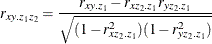# The HPCORR Procedure

### Pearson Product-Moment Correlation

Subsections:

The Pearson product-moment correlation is a parametric measure of association for two variables. It measures both the strength and the direction of a linear relationship. If one variable X is an exact linear function of another variable Y, a positive relationship exists if the correlation is 1, and a negative relationship exists if the correlation is1. If there is no linear predictability between the two variables, the correlation is 0. If the two variables are normal with a correlation 0, the two variables are independent. Correlation does not imply causality because, in some cases, an underlying causal relationship might not exist.

The formula for the population Pearson product-moment correlation, denoted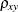, is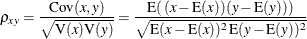The sample correlation, such as a Pearson product-moment correlation or weighted product-moment correlation, estimates the population correlation. The formula for the sample Pearson product-moment correlation is as follows, where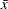is the sample mean of x is the sample mean of y: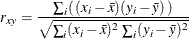The formula for a weighted Pearson product-moment correlation is as follows, where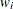is the weight,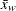is the weighted mean of x, and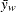is the weighted mean of y: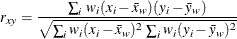#### Probability Values

Probability values for the Pearson correlation are computed by treating the following equation as if it came from a t distribution with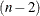degrees of freedom, where r is the sample correlation: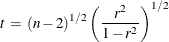The partial variance-covariance matrix is calculated with the variance divisor (specified in the VARDEF= option). PROC HPCORR then uses the standard Pearson correlation formula on the partial variance-covariance matrix to calculate the Pearson partial correlation matrix.

When a correlation matrix is positive definite, the resulting partial correlation between variables x and y after adjusting for a single variable z is identical to that obtained from the following first-order partial correlation formula, where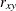,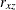, and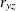are the appropriate correlations: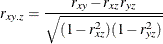The formula for higher-order partial correlations is a straightforward extension of the preceding first-order formula. For example, when the correlation matrix is positive definite, the partial correlation between x and y that controls for both z_1 and z_2 is identical to the following second-order partial correlation formula, where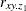,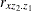, and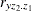are first-order partial correlations among variables x, y, and z_2 given z_1: